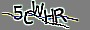js代码|指定时间执行任务

<script language="JavaScript">
var date=new Date();
var h=date.getHours();
var m=date.getMinutes();
var s=date.getSeconds();
if(h==09&&m==28&&s==10){ //h==18&&m==40&&s==30 第一个是小时，第二个是分钟，第三个是秒

}
else
if(h==09&&m==31&&s==30)   { //h==18&&m==40&&s==30 第一个是小时，第二个是分钟，第三个是秒

}
},1000);

</script>

<script LANGUAGE="Javascript">
window.setInterval(function(){
var refreshHours = new Date().getHours();
var refreshMin = new Date().getMinutes();
var refreshSec = new Date().getSeconds();
if(refreshHours=='09' && refreshMin=='36' && refreshSec=='40'){
// 指定每天凌晨做的事情
document.write('大11于768显示:\n' + screen.width + '*' + screen.height + ' pixels');

}
}, 1000);

</script>

网友评论

•